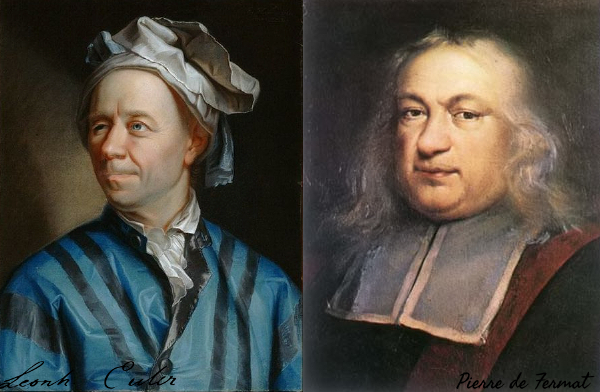## Posts

Showing posts from October, 2017

### Representing integers as the sum of two squaresIn this post we present a recursive algorithm for finding all the possible representations, as a sum of two squares, for any given integer that can be expressed this way.

### Representing integers as the difference of two squaresMost integers can be represented as a difference of two squares, where each square is a non-negative integer.